# ISEE Middle Level Math : How to find median

## Example Questions

### Example Question #91 : How To Find Median

What effect occurs on the median of a set if the lowest number of the set is decreased?

Increases

Decreases

No effect

Cannot be determined

No effect

Explanation:

Since the median is only the middle number of the set and is a countable number, changing the value of the lowest number to an even lower number has no effect on the median.  The middle number will remain the same so that means the median remains the same.

### Example Question #92 : How To Find Median

Find the median of the following data set: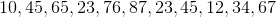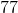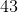Explanation:

Find the median of the following data set: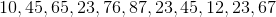First, let's reorder our data set: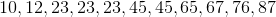Next, identify the median by choosing the middle value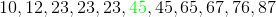### Example Question #92 : How To Find Median

Use the following data set to answer the question.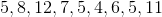Find the median of the data set.Explanation:

To find the median of a data set, we will first arrange the numbers in ascending order.  Ascending order means from smallest to largest.  Once we arrange them in ascending order, we will locate the number that is in the middle or center of the set.

So, given the setwe will first arrange them in ascending order.  We get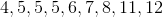Now, we will locate the number in the middle or center of the set.  We can see thatis in the center.

Therefore, the median of the data set is.

### Example Question #491 : Data Analysis

Use the following data set to answer the question.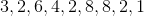Find the median of the data set.Explanation:

To find the median of a data set, we will first arrange the numbers in ascending order.  Ascending order means from smallest to largest.  Once we arrange them in ascending order, we will locate the number that is in the middle or center of the set.

So, given the setwe will first arrange them in ascending order.  We get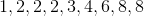Now, we will locate the number in the middle or center of the set.  We can see thatis in the center.

Therefore, the median of the data set is.

### Example Question #491 : Data Analysis

Find the median of the following data set.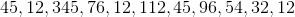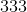Explanation:

Find the median of the following data set.To begin, put the terms in ascending order: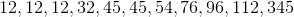Next, identify the middle term, this will be our median: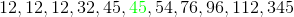Our median is therefore 45

### Example Question #491 : Data Analysis

Use the following data set to find the median: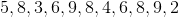Find the median.Explanation:

To find the median of a data set, we will first arrange the numbers in ascending order (from smallest to largest).  Then, we will locate the number in the middle or center of the set.

So, in the data setwe will arrange the numbers in ascending order.  We get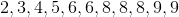Now, we will locate the number in the middle of the set.  We can see that the number in the middle is.

Therefore, the median of the data set is.

### Example Question #493 : Data Analysis

Use the following data set to answer the question: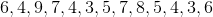Find the median.Explanation:

To find the median of a data set, we will first arrange the numbers in ascending (from smallest to largest) order, then we will locate the number in the middle of the list.

So, using the data setwe will arrange the numbers in ascending order.  We get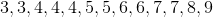Now, we will locate the number in the middle of the list.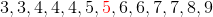We can see that it is 5.

Therefore, the median of the data set is.

### Example Question #494 : Data Analysis

Use the following data set to answer the question: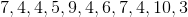Find the median.Explanation:

To find the median of a data set, we will first arrange the numbers in ascending (from smallest to largest) order.  Then, we will locate the number in the middle of the set.

So, given the setwe will first arrange them in ascending order.  We get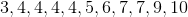Now, we will locate the number in the middle of the data set.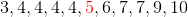We can see that it is 5.

Therefore, the median of the data set is.

### Example Question #495 : Data Analysis

Use the following data set to answer the question: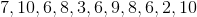Find the median.Explanation:

To find the median of a data set, we will first arrange the numbers in ascending order, then we will locate the number in the middle of the set.

So, given the data setwe will arrange the numbers in ascending order.  To do that, we will arrange them from smallest to largest.  So,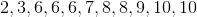Now, we will find the number that is in the middle of the set.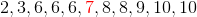We can see that it is.

Therefore, the median of the data set is.

### Example Question #496 : Data Analysis

Use the following data set to answer the question: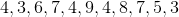Find the median.Explanation:

To find the median of a data set, we will first arrange the numbers in ascending order.  Then, we will locate the number in the middle of the set.

So, given the data setwe will first arrange the numbers in ascending order.  This simply means we will arrange them from smallest to largest.  So, we get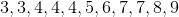Now, we will locate the number in the middle of the set.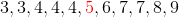We can see that it is 5.

Therefore, the median of the data set is 5.

### All ISEE Middle Level Math Resources